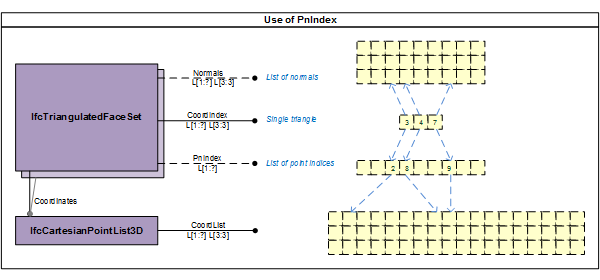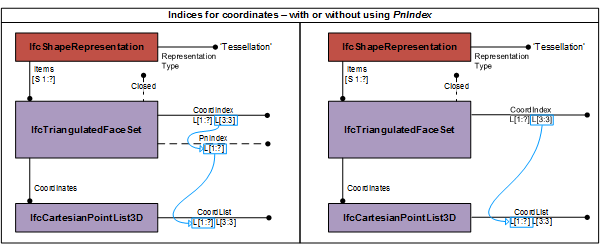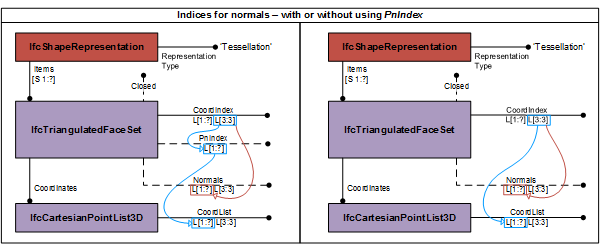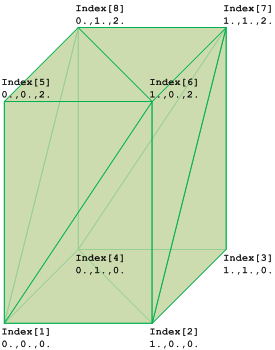# IFC 4.3.x development

## 8.8.3.46 IfcTriangulatedFaceSet

### 8.8.3.46.1 Semantic definitions at the entity

The IfcTriangulatedFaceSet is a tessellated face set with all faces being bound by triangles. The faces are constructed by implicit polylines defined by three Cartesian points. Depending on the value of the attribute Closed the instance of IfcTriangulatedFaceSet represents:

• if TRUE, a boundary represention (or B-rep);
• if FALSE, a face based surface representation.

The coordinates of each point are provided by a one-based index into an ordered list of Cartesian points provided by the two-dimensional list CoordIndex, where

• the first dimension of the two-dimensional list addresses the list of triangular faces;
• the second dimension of the two-dimensional list provides exactly three indices. Each index points to a Cartesian point being a vertex of the triangle.

Depending on the provision of PnIndex the indices point either directly into the IfcCartesianPointList3D referenced by Coordinates defined at the supertype IfcTessellatedFaceSet, or they point into the PnIndex where the integer values at that position provides the location of the coordinate values within the IfcCartesianPointList3D. See Figure 1 for the handling of point index.See Figure 2 for using the IfcTriangulatedFaceSet without (as default) and with using the PnIndex.Optionally normals can be provided. Only normals per vector are supported allowing for visually curved triangles when the three normals of the corresponding three vertices of a triangle are not co-linear to the normal of the triangular face. If they are identical, normals shall be omited.

NOTE  In case of a body representation having triangular faces with curvature, different normals for all three vertices in context of this triangles are exported, describing the curvature for smooth visualisation. In case of triangular faces with no curvature, the three vertex-based normals are identical and could always be computed from the face. Therefore vertices should only be exported to support smooth visualisation by curvature of triangles. In case of faceted representation no vertices should be exported.

Normals are provided by the two-dimensional list Normals, where

• the first dimension of the two-dimensional list addresses the corresponding list of vertex indices of the triangular faces;
• the second dimension of the two-dimensional list provides exactly three real values to determine the direction of the normal at this vertex of the triangle.

The indices in the CoordIndex point into the list of Normals. The indices of CoordIndex are applied to both points, either directly directly, or via the PnIndex and normals. See Figure 3 for handling of normals.NOTE  Using corresponding lists of points and normals allows to use only a single list of indices into both at the expense of having non-unique collections of vertices.

Figure 4 shows the use of IfcTriangulatedFaceSet without annotation. The diagram of the IfcTriangulatedFaceSet represents the indices and the ordered list into which the indices point. The index starts with 1 (indexed as 1 to N), if the greatest index in CoordIndex in N, then the IfcCartesianPointList shall have N lists of 3:3 coordinates.Figure 168 shows an IfcTriangulatedFaceSet represented by CoordIndex: ((1,6,5), (1,2,6), (6,2,7), (7,2,3), (7,8,6), (6,8,5), (5,8,1), (1,8,4), (4,2,1), (2,4,3), (4,8,7), (7,3,4)) IfcCartesianPointList3D: ((0.,0.,0.), (1.,0.,0.), (1.,1.,0.), (0.,1.,0.), (0.,0.,2.), (1.,0.,2.), (1.,1.,2.), (0.,1.,2.)) Figure 4 — Triangulated face set geometry

NOTE  The definition of IfcTriangulatedFaceSet is based on the indexedFaceSet and indexedTriangleSet defined in ISO/IEC 19775-1. The use of PnIndex has been adopted from triangulated_surface_set defined in ISO-10303-42.

HISTORY  New entity in IFC4.

Informal Propositions:

1. If Closed = TRUE, the same topological constraints as imposed by an faceted boundary representation shall be safisfied. In particular:
• each face shall be unique;
• each edge shall be used by exactly two boundaries of faces;
• the topological normal at each point on the boundary of the triangulated face set shall point away from the mater ial of the solid.

### 8.8.3.46.1 Attributes

# Attribute Type Description
IfcRepresentationItem
LayerAssignment SET [0:1] OF IfcPresentationLayerAssignment FOR AssignedItemsAssignment of the representation item to a single or multiple layer(s). The LayerAssignments can override a LayerAssignments of the IfcRepresentation it is used within the list of Items.

> IFC2x3 CHANGE  The inverse attribute LayerAssignments has been added.

> IFC4 CHANGE  The inverse attribute LayerAssignment has been restricted to max 1. Upward compatibility for file based exchange is guaranteed.

StyledByItem SET [0:1] OF IfcStyledItem FOR Item Reference to the IfcStyledItem that provides presentation information to the representation, e.g. a curve style, including colour and thickness to a geometric curve.

> IFC2x3 CHANGE  The inverse attribute StyledByItem has been added.
IfcGeometricRepresentationItem
IfcTessellatedItem
IfcTessellatedFaceSet
1 Coordinates IfcCartesianPointList3D An ordered list of Cartesian points used by the coordinate index defined at the subtypes of IfcTessellatedFaceSet.
Dim INVALID The space dimensionality of this geometric representation item, it is always 3.
HasColours SET [0:1] OF IfcIndexedColourMap FOR MappedTo Reference to the indexed colour map providing the corresponding colour RGB values to the faces of the subtypes of IfcTessellatedFaceSet.
HasTextures SET [0:?] OF IfcIndexedTextureMap FOR MappedTo Reference to the indexed texture map providing the corresponding texture coordinates to the vertices bounding the faces of the subtypes of IfcTessellatedFaceSet.
IfcTriangulatedFaceSet
2 Normals OPTIONAL LIST [1:?] OF LIST [3:3] OF IfcParameterValue An ordered list of three directions for normals. It is a two-dimensional list of directions provided by three parameter

values.

* The first dimension corresponds to the vertex indices of the Coordindex

* The second dimension has exactly three values,  the x-direction,  the y-direction and  the z-directions
3 Closed OPTIONAL IfcBoolean Indication whether the IfcTriangulatedFaceSet is a closed shell or not. If omited no such information can be provided.
4 CoordIndex LIST [1:?] OF LIST [3:3] OF IfcPositiveInteger Two-dimensional list for the indexed-based triangles, where

* The first dimension represents the triangles (from 1 to N)

* The second dimension has exactly three values representing the indices to three vertex points (from 1 to 3).

> NOTE  The coordinates of the vertices are provided by the indexed list of _SELF\IfcTessellatedFaceSet.Coordinates.CoordList_.
5 PnIndex OPTIONAL LIST [1:?] OF IfcPositiveInteger The list of integers defining the locations in the IfcCartesianPointList3D to obtain the point coordinates for the indices withint the CoordIndex. If the PnIndex is not provided the indices point directly into the IfcCartesianPointList3D.
NumberOfTrianglesINVALID Derived number of triangles used for this triangulation.

### 8.8.3.46.3 Formal representations

```ENTITY IfcTriangulatedFaceSet
SUPERTYPE OF (ONEOF
(IfcTriangulatedIrregularNetwork))
SUBTYPE OF (IfcTessellatedFaceSet);
Normals : OPTIONAL LIST [1:?] OF LIST [3:3] OF IfcParameterValue;
Closed : OPTIONAL IfcBoolean;
CoordIndex : LIST [1:?] OF LIST [3:3] OF IfcPositiveInteger;
PnIndex : OPTIONAL LIST [1:?] OF IfcPositiveInteger;
DERIVE
NumberOfTriangles : IfcInteger := SIZEOF(CoordIndex);
END_ENTITY;```